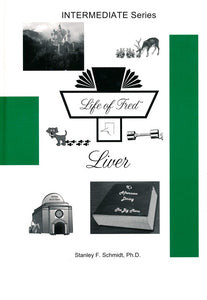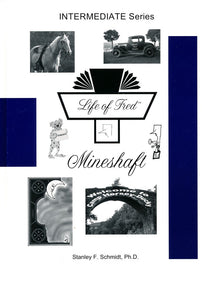# Life of Fred Intermediate Mathematics: Grades 4-5

Vendor
POLKA DOT PUBLISHING
Regular price
Sold out
Sale price
\$48.00
Quantity must be 1 or more

Life of Fred Intermediate is specially designed for students who are not yet 10 years of age but have finished the Elementary Series. This series is also is excellent for those who are in 5th or 6th Grade who are struggling with math or are switching from any other math curriculum.

We highly recommend that students in the 5th and 6th grade complete these three books before starting Life of Fred Fractions. Since Life of Fred presents math using a completely different approach, it can be beneficial for many students to back up a bit and review some of the concepts they have already covered. This helps them to get comfortable with this learning approach without them finding it too challenging. It will also give them practice with word problems which many students struggle with.

You are ready to start Life of Fred Intermediate when...
1. You can add and subtract
2. You have an understanding of multiplication and division
3. You have finished Life of Fred Ice Cream

Dr. Schmidt recommends that all students up to 4th grade start with Apples and work their way through the entire Elementary Series followed by the Intermediate Series.

There are three books in Intermediate Series, available individually or in a set:

1. Life of Fred: Kidneys (Sample Pages)

This book covers intermediate mathematics including . . .

• Milliliters
• Writing Numerals in Checks (\$4000 and Not \$40.00)
• Word Problems in Arithmetic
• 100%
• Na + Cl → NaCl
• Grams Vs. Ounces
• Functions
• Images of Elements of the Domain
• Volumes
• 115 Ounces = 7 Pounds and 3 Ounces
• Perimeters
• Definition of an Acre
• The Calculus Definition of Limit
• Scores, Dozens, and Braces
• Polar Form of Complex Numbers
• Exponents
• The Five Different Solutions to X5 = 32
• A Quarter after Two
• Point-Segment-Square-Cube-Tesseract
• Twelve Kinds of Set Theory
• The Best Time in History to Become a Mathematician
• Arithmetic Sequences and Series
• Preview of the Fundamental Theorem of Calculus
• Sigma Notation
• Descartes' Analytic Geometry

2. Life of Fred: Liver (Sample Pages)

This book covers intermediate mathematics including . . .

• 1/4 + 1/4 +1/4 = 3/4
• Elapsed Time
• The Three Angles of a Triangle Always Add to 180°
• The Pythagorean Theorem
• Finding Two-fifths of \$400,000
• Solving 2x - 7 = 11
• Pie Charts
• Six-sevenths of 413
• Short Division
• Quotients
• Secant Lines
• Changing Percents to Fractions — 68 Completely Worked out Examples
• Computing 2% of 550
• Functions, Domains, and Codomains
• Reducing Fractions
• Finding an Average.

3. Life of Fred: Mineshaft (Sample Pages)

This book covers intermediate mathematics including . . .

• Milligrams and Pounds
• Rounding Numbers
• Three-eights of 19,416
• Is a Quart less than (<) a Duck?
• What to Do with Eleven Hours of Free Time Each Day
• Six Is What Fraction of 24?
• Post Meridiem
• Exponents
• Extending the Domain of a Function
• Perpendicular Lines
• Diminutives
• Rhombus
• Can a Four-sided Figure Have Exactly Three Right Angles?
• Cardinality of Sets
• Sectors
• Inverse Operations
• the Largest Cardinal Number Isn't Six
• Ounces, Gills, and Pints
• 30% of 270
• Division by 100
• Subtracting 3 oz. From 4 lbs. 2 oz.
• Subtracting 7 Minutes 25 Seconds from 20 Minutes 10 Seconds
• Subtracting 2 Gallons 1 Quart from 4 Gallons
• Chords of a Circle
• Three Definitions of Diameter
• Subtracting 40 Feet from 9 Miles
• Fahrenheit to Celsius Conversions
• Does x < y Imply y > x?
• Converting 39 Inches into Feet
• Finding the Sale Price after Taking 40% Off
• the Image of an Element in the Domain of a Function
• 63°F = 17 2/9°C